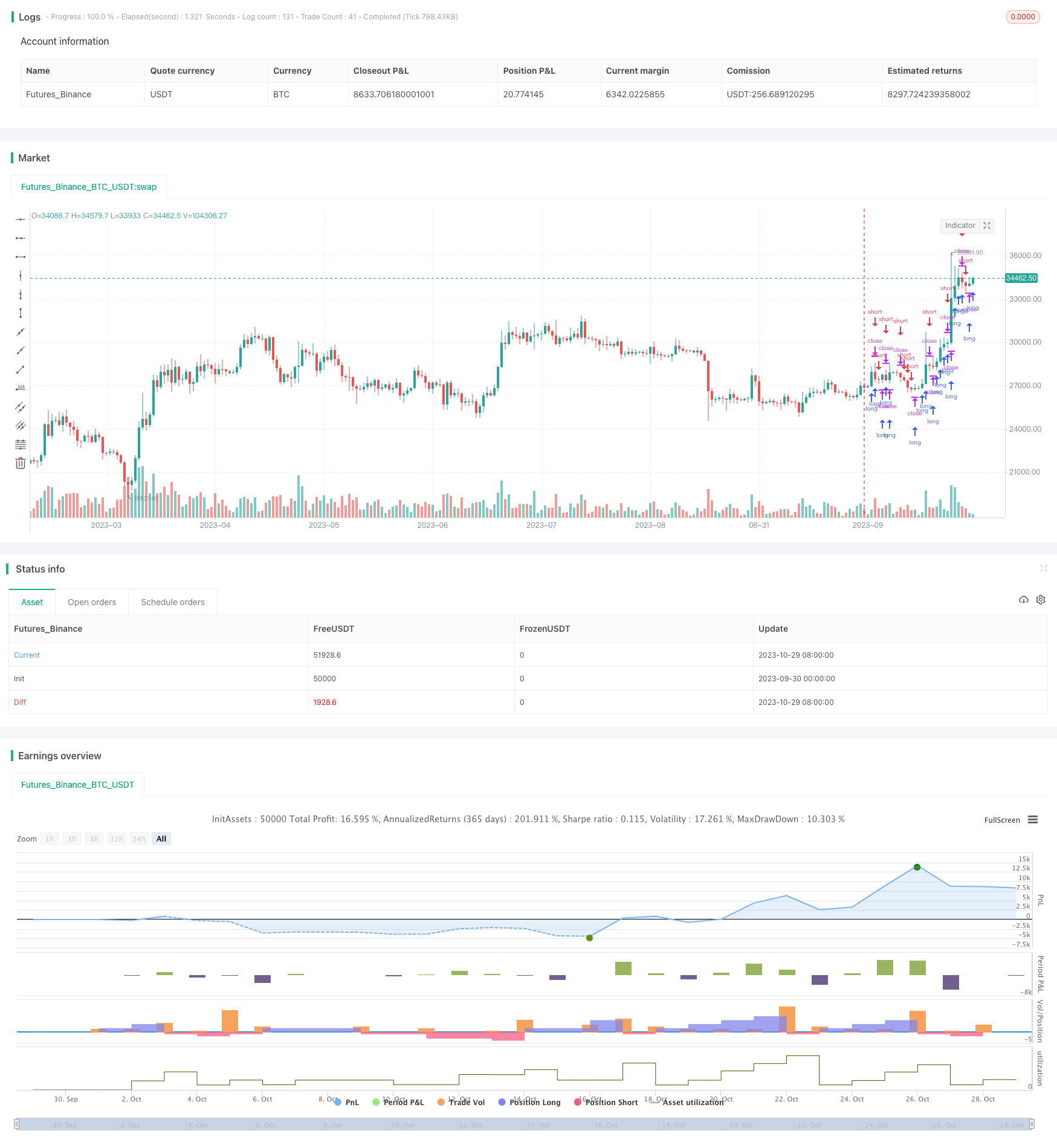# Adaptive ATR Trend Breakout Strategy

Author: ChaoZhang, Date: 2023-10-31 15:58:46
Tags:## Overview

This strategy is a trend breakout strategy based on the ATR indicator. Its main idea is to take trend breakout trades when the price exceeds a certain multiple of ATR. The strategy also includes trend confirmation and limiting trades within date ranges.

## Principle

The strategy uses the ATR indicator to measure price volatility. ATR stands for Average True Range and it measures the average volatility over a period. The length parameter in the strategy calculates the ATR period and numATRs represents the ATR multiplier for breakout.

When the price breaks out above the upper numATRs multiple of ATR, a long position is taken. When the price breaks below the lower numATRs multiple of ATR, a short position is taken.

In addition, the strategy includes needlong and needshort bool variables to control only long or only short trades. It also sets date ranges to limit trading within specified dates.

The strategy uses a size variable to determine position size and calculates order size based on percentage of account equity.

• Uses ATR to automatically adapt to market volatility without manual profit/loss settings.

• Flexible to choose long, short or long/short only.

• Can set date ranges to avoid trading at important events.

• Flexible position sizing based on account equity percentage.

## Risks and Solutions

• ATR only considers price volatility. It may have insufficient stop loss during huge market swings. Other indicators can be combined.

• Date range limits may miss opportunities if no good setups before/after. Can expand date range slightly.

• Equity percentage sizing risks large losses on single trades. Reasonable percentages needed.

## Optimization Ideas

• Add moving averages for trend filter to reduce false breakout noise trades.

• Test ATR periods to find optimal parameters.

• Combine with other strategies to utilize strengths and improve stability.

## Conclusion

This is an understandable trend following strategy using ATR to adapt to volatility. Parameter optimization and combining with other strategies can further improve performance and stability. But large single-trade losses should be avoided and insufficient stops during huge swings must be noted.

```/*backtest
start: 2023-09-30 00:00:00
end: 2023-10-30 00:00:00
period: 1d
basePeriod: 1h
exchanges: [{"eid":"Futures_Binance","currency":"BTC_USDT"}]
*/

//Noro
//2018

//@version=3
strategy(title = "Noro's Volty Strategy v1.0", shorttitle = "Volty 1.0", overlay = true, default_qty_type = strategy.percent_of_equity, default_qty_value = 100, pyramiding = 100)

//Settings
needlong = input(true, defval = true, title = "Long")
needshort = input(false, defval = false, title = "Short")
capital = input(100, defval = 100, minval = 1, maxval = 10000, title = "Lot, %")
length = input(5)
numATRs = input(0.75)
fromyear = input(1900, defval = 1900, minval = 1900, maxval = 2100, title = "From Year")
toyear = input(2100, defval = 2100, minval = 1900, maxval = 2100, title = "To Year")
frommonth = input(01, defval = 01, minval = 01, maxval = 12, title = "From Month")
tomonth = input(12, defval = 12, minval = 01, maxval = 12, title = "To Month")
fromday = input(01, defval = 01, minval = 01, maxval = 31, title = "From day")
today = input(31, defval = 31, minval = 01, maxval = 31, title = "To day")

//Indicator
atrs = sma(tr, length) * numATRs

size = strategy.position_size
lot = 0.0
lot := size == 0 ? strategy.equity / close * capital / 100 : lot
if (not na(close[length])) and needlong
strategy.entry("L", strategy.long, lot, stop = close + atrs, when = (time > timestamp(fromyear, frommonth, fromday, 00, 00) and time < timestamp(toyear, tomonth, today, 23, 59)))
if (not na(close[length])) and needlong == false
strategy.entry("L", strategy.long, 0, stop = close + atrs, when = (time > timestamp(fromyear, frommonth, fromday, 00, 00) and time < timestamp(toyear, tomonth, today, 23, 59)))
if (not na(close[length])) and needshort
strategy.entry("S", strategy.short, lot, stop = close - atrs, when = (time > timestamp(fromyear, frommonth, fromday, 00, 00) and time < timestamp(toyear, tomonth, today, 23, 59)))
if (not na(close[length])) and needshort == false
strategy.entry("S", strategy.short, 0, stop = close - atrs, when = (time > timestamp(fromyear, frommonth, fromday, 00, 00) and time < timestamp(toyear, tomonth, today, 23, 59)))
```

More